• 语谱图 基频 共振峰

万次阅读 多人点赞 2017-12-23 22:49:42
语谱图、基频、共振峰
• 窄带语谱图和宽带语谱图

首先，什么是语谱图。最通常的，就是语音短时傅里叶变换的幅度画出的2D图。之所以是通常的，是因为可以不是傅里叶变换。“窄带”，顾名思义，带宽小，则时宽大，则短时窗长，窄带语谱图就是长窗条件下画出的语谱图。“宽带”，正好相反。至于“横竖条纹”，窄带语谱图的带宽窄，那么在频率上就“分得开”，即能将语音各次谐波“看得很清楚”，即表现为“横线”。“横”就体现出了频率分辨率高。分辨率可以直观的看做“分开能力”。“频率分辨率”高就是在频率上将各次谐波分开的能力高，表现为能分辨出各次谐波的能力高，频率分辨率越高，越容易分辨各次谐波。类似的，宽带语谱图的时宽窄，那么在时间上就“分得开”，即能将语音在时间上重复的部分“看得很清楚”，即表现为“竖线”。“竖”就体现出了时间分辨率高。时间分辨率越高，谱图上的竖线看得越清楚。图1和图2分别示出了一条语音句子的窄带语谱图和宽带语谱图。短时窗长度分别是20ms和2ms。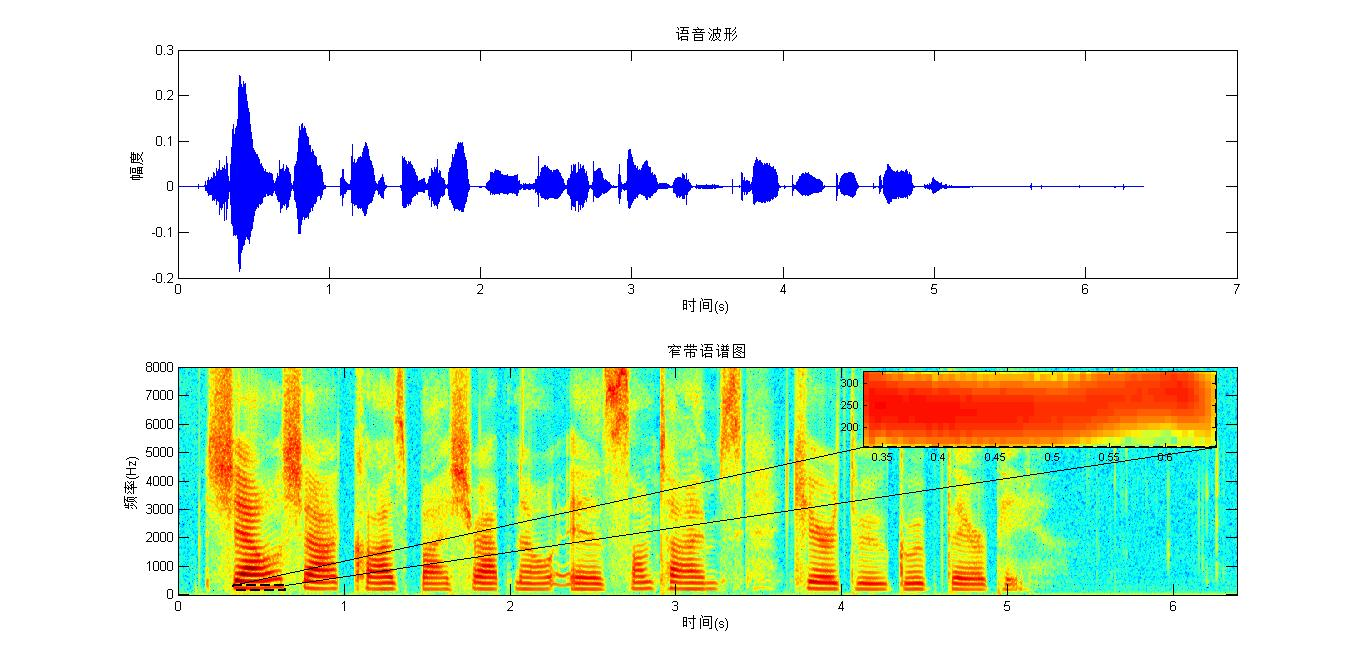图1是一条语音及其窄带语谱图。上图是语音时间波形，下图是上图的窄带语谱图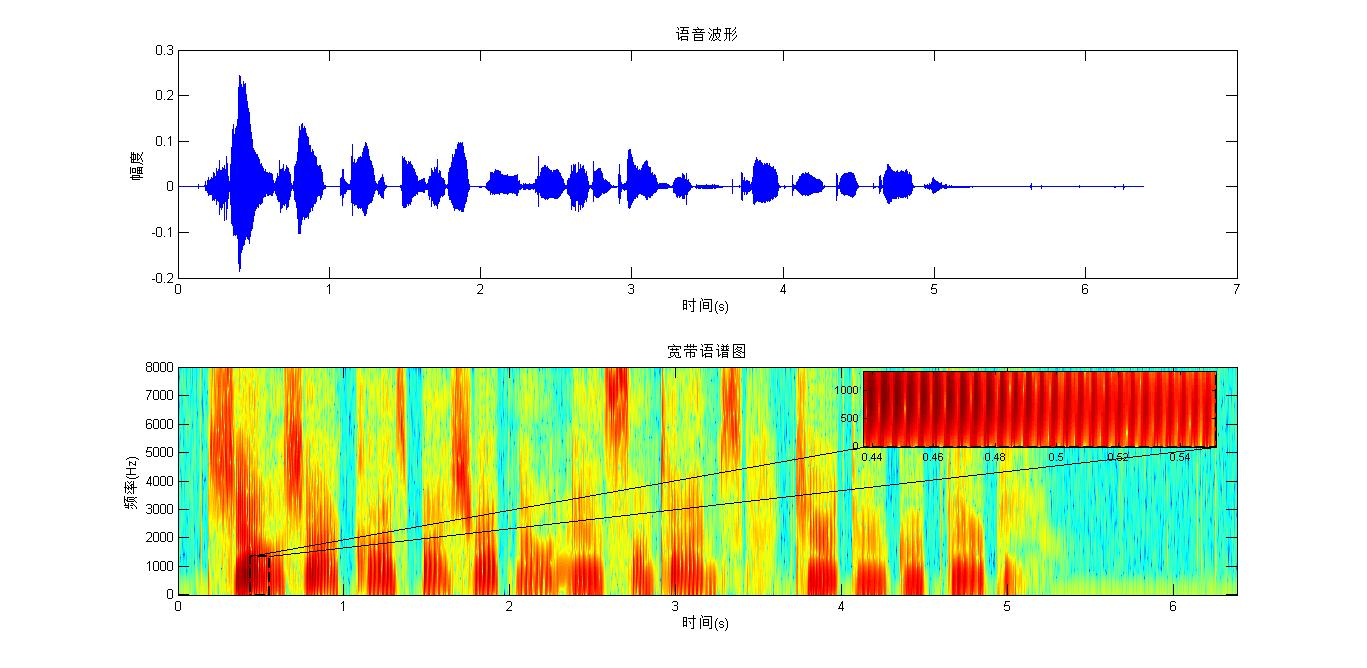图2同一条语音及其宽带语谱图。上图是语音时间波形，下图是上图的宽带语谱图

图1虚框框住的部分就是一条横条纹，整个谱图中有非常多的这种横条纹。图2虚框框住的部分，在小图中清晰的显示出了一条一条的“竖线”，被框住的有27条竖线。

• 从窄带语谱图和宽带语谱图看基音频率和共振峰

基音周期表示声带的震动周期，每隔这么长时间（震动周期），有一个气流通过，“每隔”就体现了周期性，这就是基音周期，那么谱图上就应该有这个频率的信号分量，而且这个频率的幅度（能量）不应该很小，因为每隔一段时间“就有”一团能量通过声带。所以基音频率所在的成分在窄带语谱图上应该是所有横条纹中频率范围最低的那条。在图1中，用虚线框框住的部分就表示基音频率成分，与其在同一水平线上的条纹都表示该时刻的基音频率成分，这条条纹对应的纵轴刻度值就表示基音频率。从图1小图可估计基音频率大约在250Hz左右，基音频率略有波动，0.5s处大约是240Hz。其他横条纹就是各次谐波，这些谐波中有些地方颜色比同时刻其附近其他横条纹颜色要深，这些颜色深的条纹表示共振峰。有些时刻，颜色较局部附近深的条纹不止一条，这些深色条纹组成了各次共振峰，如第一、第二、第三共振峰。图2，宽带语谱图的基音频率和共振峰就不清晰了。但是其仍可以看出基音周期，图2小图具有明显的竖线，两条竖线之间的时间就表示基音周期。在0.44s到0.54s时间段内大约有25条竖线，即24个间隔，则基音周期可估计为（0.54-0.44）/24=4.17ms，则基音频率估计为240Hz。

• 从语音时域波形上估计基音周期、频谱曲线上估计基音频率

选取0.5s处的一段语音片段，长度为20ms。其时域波形和频谱如图3。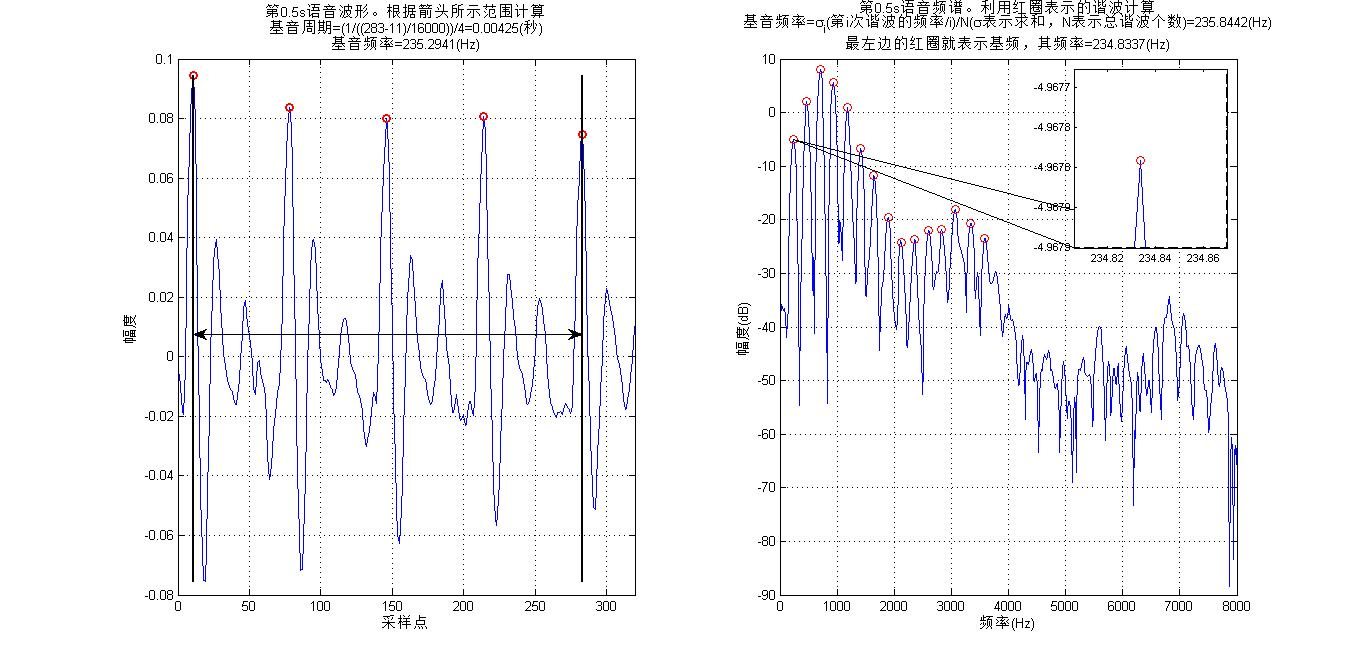图3 0.5s处语音片段时域波形及其频谱
图3左图，用红圈圈示的尖峰用于估计基音周期，双向箭头表示时间范围内5个圈共4个相似的波段，这4段就表示4个基音周期，则可估计基音周期约为4.25ms，则基音频率约为235.2941Hz。图3右图，频谱具有明显小尖峰，这些尖峰在低频部分（可认为语音频率3400Hz内）比较有规律且平滑，高频出现小幅度的“杂乱”，这些“杂乱”表示噪声，来源有录音设备及量化噪声等（虽然幅度很小，甚至根本就“听”不出来，但存在是事实）。这些尖峰就是各次谐波，从左往右一次是1次、2次、3次……谐波。其中最左边的尖峰对应基音频率，其横坐标对应的值表示基音频率，从小图可看到大约为234.83Hz。利用各次谐波可以得到更精确的估计，图中用红圈表示用于估计基频的谐波，共选取了15个，估计出基频为234.8337Hz。

• 从语音频谱曲线上看共振峰

基音频率体现的是声源的信息，而共振峰体现的是声道的信息。为便于比较和观察，将图3这个语音片段的源和系统分离，分别展示源的频谱和声道的频谱，观察一致性。图4、5显示的是源的频谱、声道的频谱、语音的频谱及共振峰的位置信息，图5去掉了小图。粉红线是源信息的频谱，红线是语音的频谱，黑色虚线是语音频谱的包络，蓝线是声道频谱，蓝色圈圈标示出声道频谱的峰值点，粉红虚竖线显示这些峰值点的位置。根据语音产生的源-滤波器模型及源、系统的卷积解释，语音频谱的包络显示的是声道的信息，而小尖峰显示源的信息，如谐波。可看到粉红线和红线二者的峰值的位置正好一一对应，体现了源的信息，而且粉红线（源）明显没有包络峰值，说明声道的信息被滤除。同样，蓝线没有了小尖峰，即滤掉了源的信息。并且声道的峰值点位置与语音频谱包络的峰值位置也正好一一对应。蓝圈圈的个数表示共振峰的个数，共有4个，从左至右分别称为F1、F2、F3、F4，（F0是基音频率）。它们横轴值表示共振峰频率值，某共振峰带宽就表示该共振峰所占频带宽度。这个图还暗示了另一个有趣的事实，源所占的频率范围和声道所占的频率范围是一样的，在频域利用高通或低通或带通的方法分离源和系统是行不通的。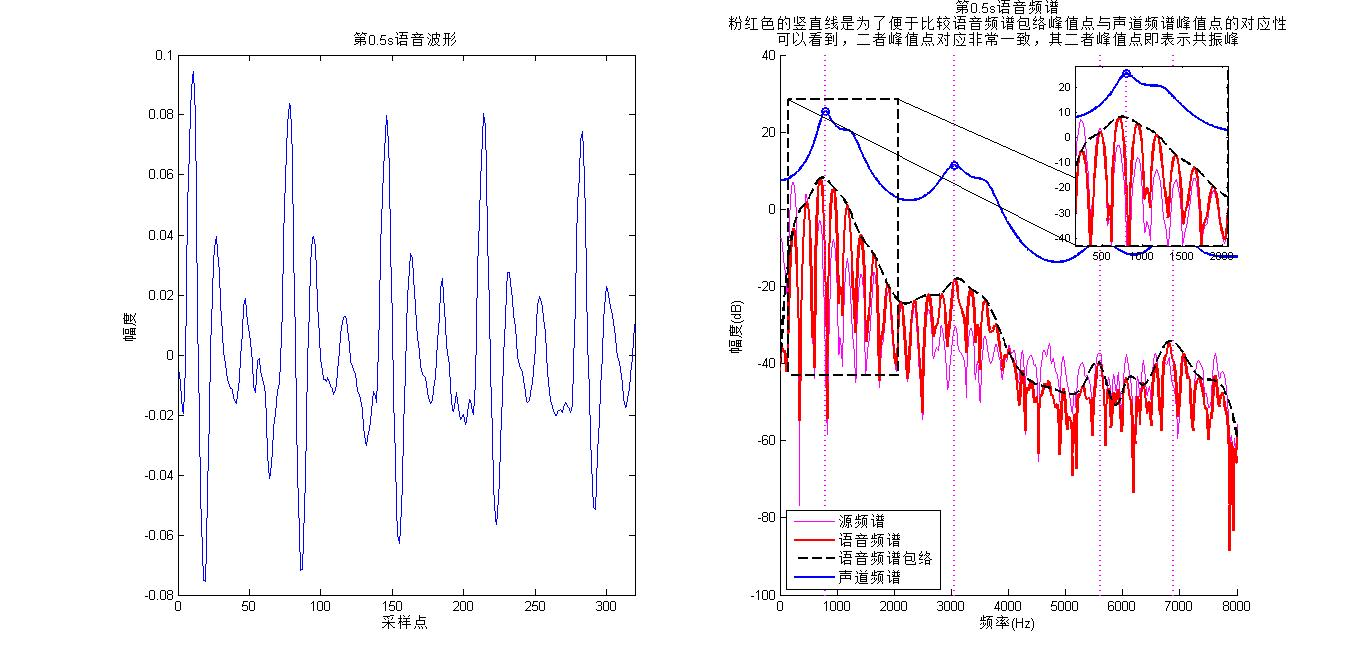图4语音片段时域波形及其源、声道和语音频谱。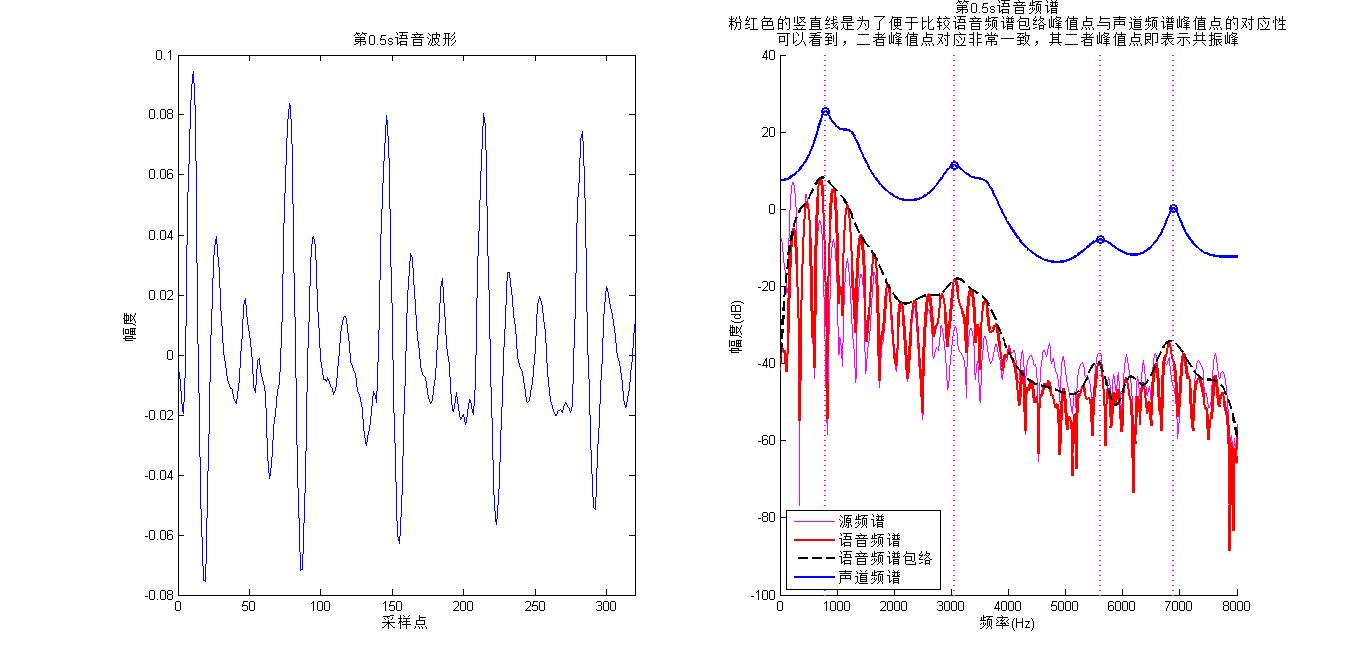图5语音片段时域波形及其源、声道和语音频谱。去掉小图（小图遮挡了部分信息）

基音频率和共振峰是能从谱图、频谱上看出来的语音的最基本的信息，当然可以看出其他更多的信息。比如，窄带语谱图上基本上可以发现，低频部分，横条纹比较直，而高频部分，条纹变“弯”了，这表示什么？图1小图可明显看到，基音频率也不是不变的，其也具有波动，我们可以人为的将基频线连接起来为一条曲线，这称为基音跟踪。共振峰表示“谐振”，频谱上表示为频谱包络（其实是上包络）的峰值，那么频谱下包络的谷值点就表示“反谐振”。频谱的“尖峰”显示的是源的信息，小尖包突起是周期性的，是有用的，那么“杂乱”的源头是非周期性的，这在语音转换中是一种很重要的信息。

PS：1、源-系统信息分离和提取参考论文：
“Glottal wave analysis with pitchsynchronous iterative adaptive inverse filtering” Paavo Alku. SpeechCommunication 11(2-3): 109-118 (1992)
2、源-系统分离代码链接：http://users.tkk.fi/~traitio/research.html

圣诞节诶，不喜欢过节，Miss总是不收敛

展开全文• 具有相同的音质 。在语音声学中，人声也同样受自身生理如鼻孔、咽腔、口腔大小的影响有自身的共振峰区。通过利用这些共鸣空间的形状和...改变共振峰可以产生所有的原音和某些辅音，在共振峰中也包含辅音的重要信息...

具有相同的音质 。

在语音声学中，人声也同样受自身生理如鼻孔、咽腔、口腔大小的影响有自身的共振峰区。通过利用这些共鸣空间的形状和大小不同的变化(例如改变咽喉、嘴形)，我们就能改变声音的共振峰。我们之所以能够区分不同的人声、元音，主要也是依靠它们的共振峰分布的位置。

共振峰反映了声道谐振特性的重要特点，它代表了发音信息的最直接的来源。改变共振峰可以产生所有的原音和某些辅音，在共振峰中也包含辅音的重要信息。人在语音感知中也利用了共振峰信息，所以共振峰已经广泛应用于语音识别的主要特征和语音编码传输的基本信息。

一个语音的共振峰模型，只用前三个时变共振峰频率就可以得到可懂度很好的合成浊音。共振峰信息包含在语音信号的频谱包络中，谱包络的峰值基本对应于共振峰频率，因此一切共振峰估计都是直接或间接地从频谱包络进行考察，关键是估计语音频谱包络。语音信号共振峰的估计，在语音信号合成、语音信号自动识别和低比特率语音信号传输等方面都起着重要作用。

如下图2.1所示利用频谱傅里叶变换相应的低频部分进行逆变换，就可以得到语音频谱的包络曲线。依据频谱包络线各峰值能量的大小可以确定出第1~第4共振峰。

图2.1 语音信号产生的离散时域模型

(二)、语音信号的预处理

语音信号从整体来看其特征及表征其本质特征的参数均是随时间而变化的，所以它是个非平稳态过程，不能用处理平稳信号的数字信号处理技术进行分析处理。但是在一个短的时间范围内(一般认为在10~30ms的短时间内)，语音信号的特性基本保持相对稳定，因而可以将其看作一个准稳态过程，即语音信号具有短时平稳性。针对这一短时特性，任何语音信号的分析和处理都要进行分帧，也就是将语音信号分为一段一段来分析其特征参数，其中每一段称为一“帧”，帧长一般取10~30ms。

信号分帧加窗处理一般采用交叠分段的方法，这是为了使帧和帧之间平滑过渡，保持其连续性。前一帧和后一帧的交叠部分称为帧移。帧移与帧长的比值一般取为0~1/2。分帧是用可移动的的有限长度窗口进行加权的方法实现的，即用一定的窗函数w(n)来乘s(n)，从而形成加窗语音信号Sw (n)= s(n)* w (n)。

在语音信号处理中常用的窗函数是矩形窗和汉明窗等，他们的表达式如下(其中N为帧长)：

矩形窗： (2-1)

汉明窗： (2-2)

窗函数w(n)的选择(形状和长度)，对于短时分析参数的特性有重要影响。为此应选择合适的窗口，使其短时参数能更好地反映语音信号的特性变化。数字信号处理理论告诉我们，两个信号的时域相乘，在频域相卷积，矩形信号频谱高频成分必将影响语音信号的高频部分，一般用高频分量幅度较小的窗形，以避免这些影响。汉明(Hamming)窗的带宽是矩形窗的两倍，但带外衰减却比矩形窗大得多。这样看来，根据处理的要求，只要以不影响或少影响处理需要的语音特性为标准来选择窗形较为适宜。本文在进行共振峰检测时主要采用汉明窗和矩形窗，窗长视帧长和采样频率而定。

原文链接:http://www.51jrft.com/dzxx/gdxx/1498.html

展开全文• 语音共振峰的获取python

千次阅读 2020-11-28 14:42:46
2 共振峰的获取 2.1 倒谱法求共振峰 流程如下： #mermaid-svg-OSRurQA7CLZuLbZe .label{font-family:'trebuchet ms', verdana, arial;font-family:var(--mermaid-font-family);fill:#333;color:#333}#mermaid-svg-...

2 共振峰的获取

2.1 倒谱法求共振峰

流程如下：

具体步骤：

倒谱法共振峰估计的算法过程为：

• 对语音信号 x x 进行预加重，并进行加窗，FFT处理
X ( k ) = ∑ n = 1 N x ( n ) e − 2 π k n j N (2-1) X(k)=\sum_{n=1}^Nx(n)e^{-\frac{2\pi kn j}{N}} \tag{2-1}
窗函数为汉明窗，长度为320。声音采样频率为8000Hz，FFT长度默认为65536。

• X ( k ) X(k) 的倒谱：
x ^ ( n ) = 1 N ∑ k = 1 N lg ⁡ ∣ X ( k ) ∣ e − 2 π k n j N (2-2) \hat x(n)=\frac{1}{N}\sum_{k=1}^N\lg|X(k)|e^{-\frac{2\pi kn j}{N}} \tag{2-2}

• 给倒谱信号加窗得： h ( n ) = x ^ ( n ) × h ( n ) h(n)=\hat x(n)\times h(n) ,这里的窗函数和倒谱的分辨率有关（和采样率和FFT长度有关）：
h ( n ) = { 1 n ⩽ n 0 − 1 & n ⩾ N − n 0 + 1 0 n 0 − 1 < n < N − n 0 + 1 , n ∈ [ 0 , N − 1 ] (2-3) h(n)=\left \{\begin{array}{lc} 1&n\leqslant n_0-1 \&n\geqslant N-n_0+1\\ 0& n_0-1<n<N-n_0+1 \end{array}\right.,n\in[0,N-1] \tag{2-3}
式中 n 0 n_0 是窗函数的宽度

• 求包络线

• 在包络线上寻找极大值，获得相应共振峰参数。

实现代码为：

def Formant_Cepst(u, cepstL):
"""
倒谱法共振峰估计函数
:param u:输入信号
:param cepstL:🔪频率上窗函数的宽度
:return: val共振峰幅值
:return: loc共振峰位置
:return: spec包络线
"""
wlen2 = len(u) // 2
u_fft=np.fft.fft(u)                         #按式（2-1）计算
U = np.log(np.abs( u_fft[:wlen2]))
Cepst = np.fft.ifft(U)                      #按式（2-2）计算
cepst = np.zeros(wlen2, dtype=np.complex)
cepst[:cepstL] = Cepst[:cepstL]             #按式（2-3）计算
cepst[-cepstL + 1:] = Cepst[-cepstL + 1:]	#取第二个式子的相反
spec = np.real(np.fft.fft(cepst))
val, loc = local_maxium(spec)               #在包络线上寻找极大值
return val, loc, spec

2.2 测试结果

低通窗函数 n 0 = 7 n_0=7 。测试男性a1画出波形如图所示：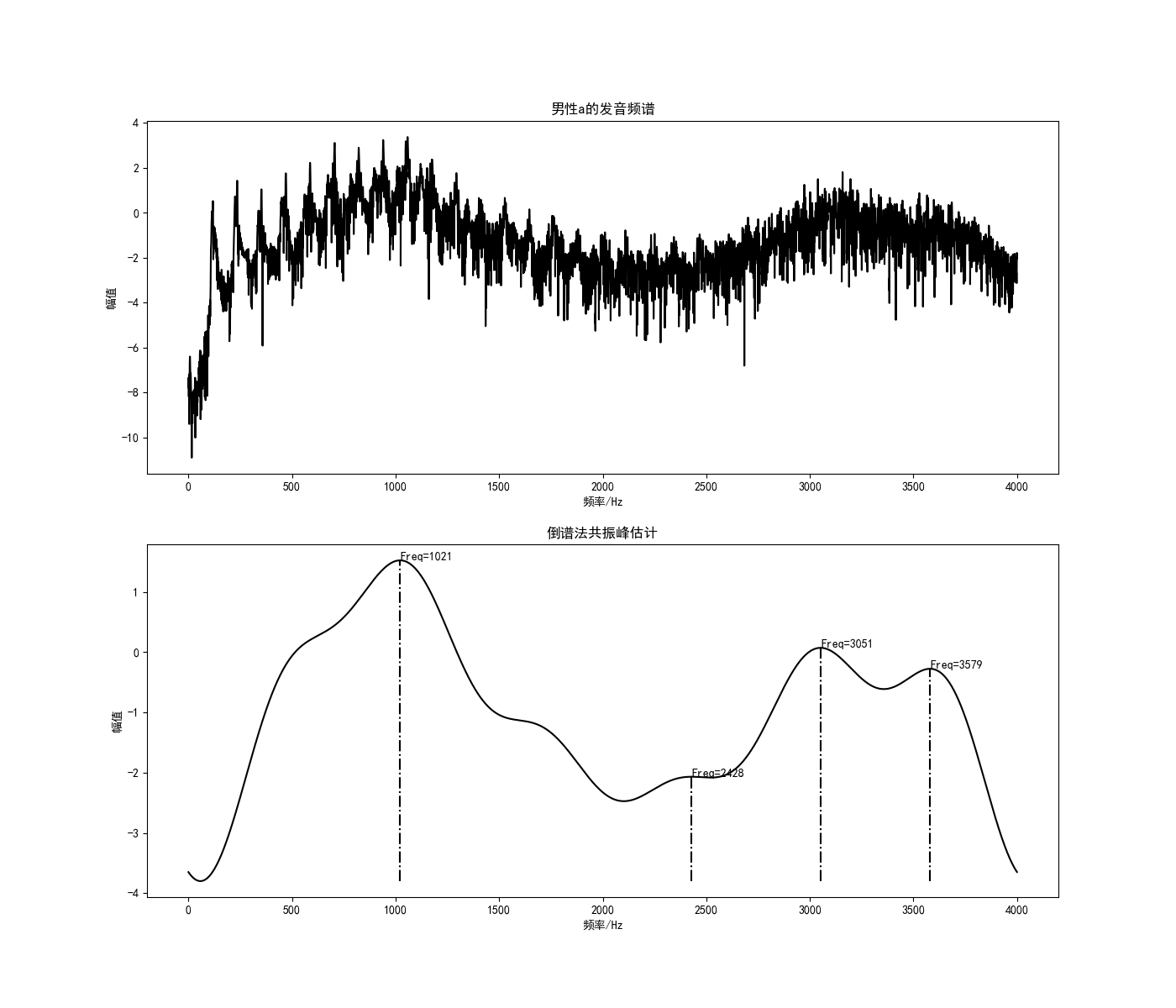五元音的前两共振峰的测试结果如下：

元音第一共振峰第二共振峰
a1/a21021/10442428/2404
o1/o2596/5221583/1673
e1/e2469/408996/1018
i1/i2372/3751149/1031
u1/u2508/5141543/3494

2.3 实现程序

CepstrumFormant.py

from 共振峰估计函数 import *
from scipy.signal import lfilter
import librosa
import numpy as np
import matplotlib.pyplot as plt

plt.figure(figsize=(14, 12))
path="F:\\python\\VowelStuday\\SingleVowel\\aoeiu元音音频\\原始元音男性\\a1.wav"
#path="C4_3_y.wav"
data, fs = librosa.load(path, sr=None, mono=False)#sr=None声音保持原采样频率， mono=False声音保持原通道数
# 预处理-预加重
u = lfilter([1, -0.99], , data)

cepstL = 7
wlen = len(u)
wlen2 = wlen // 2
print("帧长={}".format(wlen))
print("帧移={}".format(wlen2))
# wlen = 256
# wlen2 = 256//2
# 预处理-加窗
u2 = np.multiply(u, np.hamming(wlen))
# 预处理-FFT,取对数 获得频域图像 取一半
U_abs = np.log(np.abs(np.fft.fft(u2))[:wlen2])
# 4.3.1
freq = [i * fs / wlen for i in range(wlen2)]
#print(freq)
#val共振峰幅值 loc共振峰位置 spec包络线
val, loc, spec = Formant_Cepst(u, cepstL)
plt.subplot(2, 1, 1)
plt.plot(freq, U_abs, 'k')
plt.xlabel('频率/Hz')           #设置x，y轴的标签
plt.ylabel('幅值')
plt.title('男性a的发音频谱')
plt.subplot(2, 1, 2)
plt.plot(freq, spec, 'k')
plt.xlabel('频率/Hz')           #设置x，y轴的标签
plt.ylabel('幅值')
plt.title('倒谱法共振峰估计')
for i in range(len(loc)):
plt.subplot(2, 1, 2)
plt.plot([freq[loc[i]], freq[loc[i]]], [np.min(spec), spec[loc[i]]], '-.k')
plt.text(freq[loc[i]], spec[loc[i]], 'Freq={}'.format(int(freq[loc[i]])))

plt.savefig('images/共振峰估计.png')
plt.show()
plt.close()

2.3 共振峰位置坐标

以元音的第一共振峰频率为x轴，第二共振峰频率为y轴，将五个元音的前两个共振峰画在一个二维空间中。五个元音是同一个人的发音。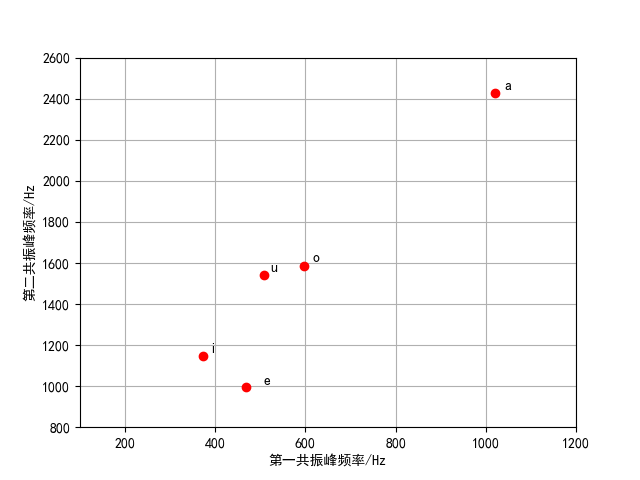2.4 多种情况下的共振峰测试

对于同一个元音，不同性别的发声人在第1共振峰频率和第2共振峰频率的两共振峰的测试结果如下：

元音第一共振峰第二共振峰
男性a发音10212428
女性a发音11452364
男性e发音469996
女性e发音5001118
男性i发音3721149
女性i发音3791106
男性o发音5961583
女性o发音6171663
男性u发音5081543
女性u发音4372426

对于同一个元音，不同性别的发声人在第1共振峰频率和第2共振峰频率的差值如下：

元音第一共振峰第二共振峰
a发音男女的差值12464
e发音男女的差值31122
i发音男女的差值743
o发音男女的差值2180
u发音男女的差值71883

从上表可以看出，对于同一个元音，不同性别的发声人在第1共振峰频率的差值控制在200Hz以内，而第1共振峰频率的差值变换幅度较大。

对于同一个元音，不同的发声人（同一性别）在第1共振峰频率和第2共振峰频率的两共振峰的测试结果如下：

元音第一共振峰第二共振峰
01号发声人a发音10212428
02号发声人a发音10632947
03号发声人a发音10981849

从上表对于同一个元音，不同的发声人（同一性别）在第1共振峰频率的差距不上特别明显，相差大约在40Hz左右，而在第2共振峰频率上的差距就很明显。

展开全文信号处理
• 当声门处准周期脉冲激励进入声道时会引起共振特性，产生一组共振频率，这一组共振频率称为共振峰频率或简称为共振峰共振峰参数包括共振峰频率、频带宽度和幅值，共振峰信息包含在语音频谱的包络中。因此共振峰参数...

《语音信号处理试验教程》（梁瑞宇等）的代码主要是Matlab实现的，现在Python比较热门，所以把这个项目大部分内容写成了Python实现，大部分是手动写的。使用CSDN博客查看帮助文件：

代码可在Github上下载busyyang/python_sound_open

声道可以被看成一根具有非均匀截面的声管，在发音时将起共鸣器的作用。当声门处准周期脉冲激励进入声道时会引起共振特性，产生一组共振频率，这一组共振频率称为共振峰频率或简称为共振峰。共振峰参数包括共振峰频率、频带宽度和幅值，共振峰信息包含在语音频谱的包络中。因此共振峰参数提取的关键是估计语音频谱包络，并认为谱包络中的最大值就是共振峰。利用语音频谱傅里叶变换相应的低频部分进行逆变换，就可以得到语音频谱的包络曲线。依据频谱包络线各峰值能量的大小确定出第1-4共振峰.

在经典的语音信号模型中，共振峰等效为声道传输函数的复数极点对。对平均长度约为17cm声道(男性) ，在3kHz范围内大致包含三个或四个共振峰，而在5 kHz范围内包含四个或五个共振峰。高于5kHz的语音信号，能量很小。浊音信号最主要的是前三个共振峰。因此一切共振峰估计都是直接或间接地对频谱包络进行考察，关键是估计语音频谱包络，并认为谱包络中的最大值就是共振峰。

共振峰估计预处理

在估计之前需要进行预加重，对信号进行高频提升，还原声门的信号。
s ′ ( n ) = s ( n ) − a ⋅ s ( n − 1 ) s'(n)=s(n)-a·s(n-1)

预加重有两个作用：

• 增加一个零点：抵消声门脉冲引起的高端频谱幅度下跌，使信号频谱变得平坦且各共振峰幅度相接近;语音中只剩下声道部分的影响，所提取的特征更加符合原声道的模型。
• 会削减低频信息，使有些基频幅值变大时，通过预加重后降低基频对共振峰检测的干扰，有利于共振峰的检测;同时减少频谱的动态范围。

另外，共振峰检测一般是分析韵母部分，所以还需要进行端点检测。可以使用基音周期检测相同的端点检测方法。

倒谱法共振峰估计

倒谱法共振峰估计的算法过程为：

• 对语音信号 x ( i ) x(i) 进行预加重，并进行加窗，分帧，FFT处理
X i ( k ) = ∑ n = 1 N x i ( n ) e − 2 π k n j N X_i(k)=\sum_{n=1}^Nx_i(n)e^{-\frac{2\pi kn j}{N}}
• X i ( k ) X_i(k) 的倒谱：
x ^ i ( n ) = 1 N ∑ k = 1 N lg ⁡ ∣ X i ( k ) ∣ e − 2 π k n j N \hat x_i(n)=\frac{1}{N}\sum_{k=1}^N\lg|X_i(k)|e^{-\frac{2\pi kn j}{N}}
• 给倒谱信号加窗得： h i ( n ) = x ^ i ( n ) × h ( n ) h_i(n)=\hat x_i(n)\times h(n) ,这里的窗函数和倒谱的分辨率有关（和采样率和FFT长度有关）：
h ( n ) = { 1 n ⩽ n 0 − 1 & n ⩾ N − n 0 + 1 0 n 0 − 1 < n < N − n 0 + 1 , n ∈ [ 0 , N − 1 ] h(n)=\left \{\begin{array}{lc} 1&n\leqslant n_0-1 \&n\geqslant N-n_0+1\\ 0& n_0-1<n<N-n_0+1 \end{array}\right.,n\in[0,N-1]
• h i ( n ) h_i(n) 的包络线： H i ( k ) = ∑ n = 1 N h i ( n ) e − 2 π k n j N H_i(k)=\sum\limits_{n=1}^{N}h_i(n)e^{-\frac{2\pi kn j}{N}}
• 在包络线张寻找极大值，获得相应共振峰参数。

LPC法共振峰估计

简化的语音产生模型是将辐射、声道以及声门激励的全部效应简化为一个时变的数字滤波器来等效，其传递函数为
H ( z ) = S ( z ) U ( z ) = G 1 − ∑ i = 1 p a i z i − 1 H(z)=\frac{S(z)}{U(z)}=\frac{G}{1-\sum\limits_{i=1}^pa_iz_i^{-1}}

z − 1 = exp ⁡ ( − j 2 π f / f s ) z^{-1}=\exp(-j2\pi f/f_s) ，则功率谱 P ( f ) P(f) 为：
P ( f ) = ∣ H ( f ) ∣ 2 = G 2 ∣ 1 − ∑ i = 1 p a i exp ⁡ ( − j 2 π i f / f s ) ∣ 2 P(f)=|H(f)|^2=\frac{G^2}{|1-\sum\limits_{i=1}^pa_i\exp(-j2\pi if/f_s)|^2}

利用FFT方法可对任意频率求得其功率谱幅值响应，并从幅值响应中找到共振峰，相应的求解方法有两种:抛物线内插法和线性预测系数求复数根法。

抛物线内插法

在任意共振峰频率 F i F_i 的局部峰值频率 m Δ f m\Delta f ( Δ f \Delta f 为谱图的频率间隔)，以及邻近的两个频率点 ( m − 1 ) Δ f (m-1)\Delta f , ( m + 1 ) Δ f (m+1)\Delta f ，以及他们的幅值分别为 H ( m − 1 ) , H ( m ) , H ( m + 1 ) H(m-1),H(m),H(m+1) ,可以用二次方程 a λ 2 + b λ + c a\lambda^2+b\lambda+c 来计算，求出更精确的中心频率 F i F_i 和带宽 B i B_i
为了方便计算，令 m Δ f m\Delta f 处为零，对应于 Δ f , 0 , + Δ f \Delta f,0,+\Delta f 处的功率谱分别为 H ( m − 1 ) , H ( m ) , H ( m + 1 ) H(m-1),H(m),H(m+1) ,由 H = a λ 2 + b λ + c H=a\lambda^2+b\lambda+c 有：
{ H ( m − 1 ) = a Δ 2 − b Δ + c H ( m ) = c H ( m + 1 ) = a Δ 2 + b Δ + c \left \{\begin{array}{ll} H(m-1)=a\Delta^2-b\Delta+c\\ H(m)=c\\ H(m+1)=a\Delta^2+b\Delta+c \end{array}\right.

假设 Δ f = 1 \Delta f=1 ,则计算系数为：
{ a = H ( m − 1 ) + H ( m + 1 ) 2 − H ( m ) b = H ( m − 1 ) + H ( m + 1 ) 2 c = H ( m ) \left \{\begin{array}{ll} a=\frac{H(m-1)+H(m+1)}{2}-H(m)\\ b=\frac{H(m-1)+H(m+1)}{2}\\ c=H(m) \end{array}\right.

极大值处中心频率为: λ m a x = − b / 2 a \lambda_{max}=-b/2a ,实际共振峰中心频率为： F i = λ m a x Δ f + m Δ f F_i=\lambda_{max}\Delta f+m\Delta f ，中心频率对应的功率谱 H p H_p 为：
H p = a λ p 2 + b λ p + c = − b 2 4 a + c H_p=a\lambda_p^2+b\lambda_p+c=-\frac{b^2}{4a}+c

求带宽，就是在某个 λ \lambda 处，使得谱值为最大值的一半：
a λ 2 + b λ + c H p = 1 2 \frac{a\lambda^2+b\lambda+c}{H_p}=\frac{1}{2}

解这个二次方程可以得到：
λ r o o t = − b ± b 2 − 4 a ( c − 0.5 H p ) 2 a \lambda _{root}=\frac{-b±\sqrt{b^2-4a(c-0.5H_p)}}{2a}

实际带宽可以写成：
B i = 2 λ b Δ f B_i=2\lambda_b\Delta f

其中 λ b = − b 2 − 4 a ( c − 0.5 H p ) 2 a \lambda_b=-\frac{b^2-4a(c-0.5H_p)}{2a}

线性预测系数求根法

预测误差滤波器表示为：
A ( z ) = 1 − ∑ i = 1 p a i z − i A(z)=1-\sum_{i=1}^pa_iz^{-i}

其多项式复根可以精确表示共振峰的中心频率与带宽。设 z i = r i e j θ i z_i=r_ie^{j\theta_i} 为任意复根，其共轭值 z i ∗ = r i e − j θ i z^*_i=r_ie^{-j\theta_i} 也是根，设 z i z_i 对应的共振峰频率为 F i F_i ，3dB带宽为 B i B_i ，那么有：
{ 2 π F i / f s = θ i e − B i π / f s − r i \left \{\begin{array}{ll} 2\pi F_i/f_s=\theta_i\\e^{-B_i\pi/f_s}-r_i \end{array} \right.

所以：
{ F i = θ i f s / 2 π B i = − ln ⁡ r i ⋅ f s / π \left \{\begin{array}{ll} F_i=\theta_if_s/2\pi\\B_i=-\ln r_i·f_s/\pi \end{array} \right.

因为预测误差滤波器阶数p是预先设定的，所以复共辄对的数量最多是p/2。因为不属于共振峰的额外极点的带宽远大于共振峰带宽，所以比较容易剔除非共振峰极点。

# 共振峰估计函数
import numpy as np
from chapter3_分析实验.timefeature import *
from chapter3_分析实验.lpc import lpc_coeff

def local_maxium(x):
"""
求序列的极大值
:param x:
:return:
"""
d = np.diff(x)
l_d = len(d)
maxium = []
loc = []
for i in range(l_d - 1):
if d[i] > 0 and d[i + 1] <= 0:
maxium.append(x[i + 1])
loc.append(i + 1)
return maxium, loc

def Formant_Cepst(u, cepstL):
"""
倒谱法共振峰估计函数
:param u:
:param cepstL:
:return:
"""
wlen2 = len(u) // 2
U = np.log(np.abs(np.fft.fft(u)[:wlen2]))
Cepst = np.fft.ifft(U)
cepst = np.zeros(wlen2, dtype=np.complex)
cepst[:cepstL] = Cepst[:cepstL]
cepst[-cepstL + 1:] = Cepst[-cepstL + 1:]
spec = np.real(np.fft.fft(cepst))
val, loc = local_maxium(spec)
return val, loc, spec

def Formant_Interpolation(u, p, fs):
"""
插值法估计共振峰函数
:param u:
:param p:
:param fs:
:return:
"""
ar, _ = lpc_coeff(u, p)
U = np.power(np.abs(np.fft.rfft(ar, 2 * 255)), -2)
df = fs / 512
val, loc = local_maxium(U)
ll = len(loc)
pp = np.zeros(ll)
F = np.zeros(ll)
Bw = np.zeros(ll)
for k in range(ll):
m = loc[k]
m1, m2 = m - 1, m + 1
p = val[k]
p1, p2 = U[m1], U[m2]
aa = (p1 + p2) / 2 - p
bb = (p2 - p1) / 2
cc = p
dm = -bb / 2 / aa
pp[k] = -bb * bb / 4 / aa + cc
m_new = m + dm
bf = -np.sqrt(bb * bb - 4 * aa * (cc - pp[k] / 2)) / aa
F[k] = (m_new - 1) * df
Bw[k] = bf * df
return F, Bw, pp, U, loc

def Formant_Root(u, p, fs, n_frmnt):
"""
LPC求根法的共振峰估计函数
:param u:
:param p:
:param fs:
:param n_frmnt:
:return:
"""
ar, _ = lpc_coeff(u, p)
U = np.power(np.abs(np.fft.rfft(ar, 2 * 255)), -2)
const = fs / (2 * np.pi)
rts = np.roots(ar)
yf = []
Bw = []
for i in range(len(ar) - 1):
re = np.real(rts[i])
im = np.imag(rts[i])
fromn = const * np.arctan2(im, re)
bw = -2 * const * np.log(np.abs(rts[i]))
if fromn > 150 and bw < 700 and fromn < fs / 2:
yf.append(fromn)
Bw.append(bw)
return yf[:min(len(yf), n_frmnt)], Bw[:min(len(Bw), n_frmnt)], U

from chapter2_基础.soundBase import *
from chapter4_特征提取.共振峰估计 import *
from scipy.signal import lfilter

plt.figure(figsize=(14, 12))

# 预处理-预加重
u = lfilter([1, -0.99], , data)

cepstL = 6
wlen = len(u)
wlen2 = wlen // 2
# 预处理-加窗
u2 = np.multiply(u, np.hamming(wlen))
# 预处理-FFT,取对数
U_abs = np.log(np.abs(np.fft.fft(u2))[:wlen2])
# 4.3.1
freq = [i * fs / wlen for i in range(wlen2)]
val, loc, spec = Formant_Cepst(u, cepstL)
plt.subplot(4, 1, 1)
plt.plot(freq, U_abs, 'k')
plt.title('频谱')
plt.subplot(4, 1, 2)
plt.plot(freq, spec, 'k')
plt.title('倒谱法共振峰估计')
for i in range(len(loc)):
plt.subplot(4, 1, 2)
plt.plot([freq[loc[i]], freq[loc[i]]], [np.min(spec), spec[loc[i]]], '-.k')
plt.text(freq[loc[i]], spec[loc[i]], 'Freq={}'.format(int(freq[loc[i]])))
# 4.3.2
p = 12
freq = [i * fs / 512 for i in range(256)]
F, Bw, pp, U, loc = Formant_Interpolation(u, p, fs)

plt.subplot(4, 1, 3)
plt.plot(freq, U)
plt.title('LPC内插法的共振峰估计')

for i in range(len(Bw)):
plt.subplot(4, 1, 3)
plt.plot([freq[loc[i]], freq[loc[i]]], [np.min(U), U[loc[i]]], '-.k')
plt.text(freq[loc[i]], U[loc[i]], 'Freq={:.0f}\nHp={:.2f}\nBw={:.2f}'.format(F[i], pp[i], Bw[i]))

# 4.3.3

p = 12
freq = [i * fs / 512 for i in range(256)]

n_frmnt = 4
F, Bw, U = Formant_Root(u, p, fs, n_frmnt)

plt.subplot(4, 1, 4)
plt.plot(freq, U)
plt.title('LPC求根法的共振峰估计')

for i in range(len(Bw)):
plt.subplot(4, 1, 4)
plt.plot([freq[loc[i]], freq[loc[i]]], [np.min(U), U[loc[i]]], '-.k')
plt.text(freq[loc[i]], U[loc[i]], 'Freq={:.0f}\nBw={:.2f}'.format(F[i], Bw[i]))

plt.savefig('images/共振峰估计.png')
plt.close()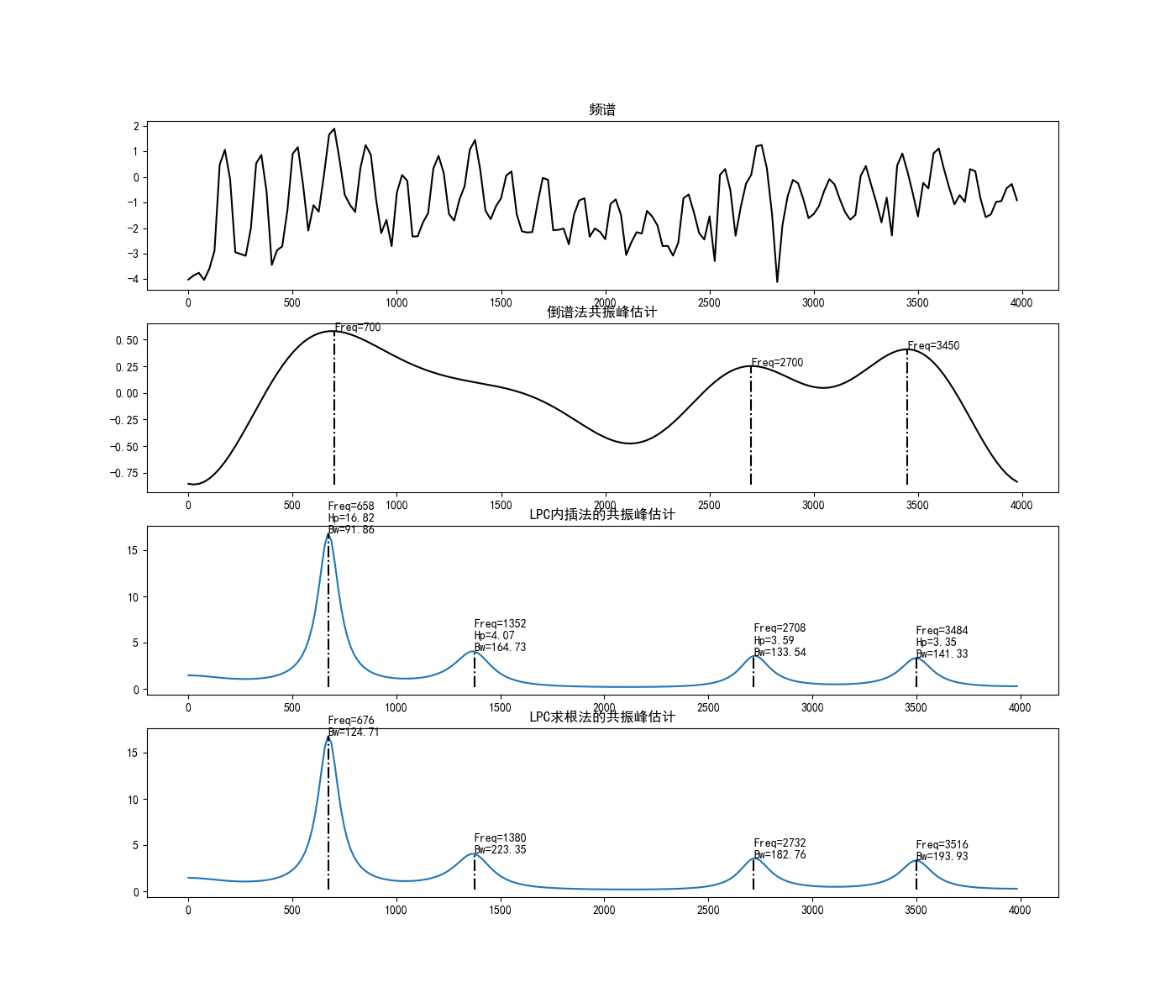展开全文python 语音识别
• 语音信号处理共振峰

千次阅读 2019-10-31 15:39:27
它们横轴值表示共振峰频率值，某共振峰带宽就表示该共振峰所占频带宽度。这个图还暗示了另一个有趣的事实，源所占的频率范围和声道所占的频率范围是一样的，在频域利用高通或低通或带通的方法分离源和系统是行不通的...
• 共振峰是指在声音的频谱中能量相对集中的一些区域，共振峰不但是音质的决定因素，而且反映了声道（共振腔）的物理特征。 元音和响辅音声谱包络曲线上的峰巅位置。共振峰的本义是指声腔的共鸣频率。在元音和响辅音的...matlab 语音识别
• 什么叫共振峰？

千次阅读 2018-08-21 19:56:20
共振峰是指在声音的频谱中能量相对集中的一些区域，共振峰不但是音质的决定因素，而且反映了声道（共振腔）的物理特征。 元音和响辅音声谱包络曲线上的峰巅位置。共振峰的本义是指声腔的共鸣频率。在元音和响辅音的...
• 我用自己的语音，读出来之后就 出现 Index ...我的Fs=22050，我想请问共振峰的个数与什么有关>> [x, fs, nbits]=wavread('C:\Users\WEICHANGCHENG\Desktop\H=15\无裂颖\1.wav'); % 读入一帧数据u=filter([...
• 通过深入分析语音信号的时域和频域性质针对语音信号幅度谱的特征设计了一种有效的基频 和共振峰提取算法并对实际语音信号进行参数提取测试实验结果证明了这种算法能够准确提取不同讲话者和录音条件下的语音
• 线性预测编码在并发元音共振峰分析中的应用 该项目着重于理解噪声对单个元音和同时元音的共振峰表示的影响。 用于此目的的数据集包括两个基本频率分别为100 Hz和126 Hz的单个元音和同时元音的值。 借助线性预测编码...
• 本文采用傅里叶变换法结合matlab讨论了一段语音信号的短时谱、语谱图、倒谱和复制谱等特征,给出了基音周期及共振峰等仿真结果。2 1年 8月 01湖北第二师范学院学报J un lo b iUmv mi o u a o o r a fHu e e￣ fEd ...
• 2011年8月第28卷第8期湖北第二师范学院学报JournalofHubeiUniversityofEducationAug．2011Vol．28No.8基于MATLAB分析语音信号频域特征肖正安(湖北第二师范学院物理与电子信息学院，武汉430205)摘要：语音信号的频域...
• 频域上表现为频率集中在低频区域,共振峰较为明显,短时能量较高。 （2）清音S 时域分析： 时域波形没有周期性，整体振幅小于浊音。 频域分析： 频域波形平缓，无共振峰，能量比较集中。 （3）爆破音t 时域分析： ...
• 现在来分析最后一块g723中最后一个未...共振峰后置滤波器 涉及函数 Spf Scale 观察itu 3.8 式49.3:  10  1 - Σ ai * λ1^i * z^(-i)  i=1 F(z)= --------------------------- (1 - 0.25 * k * z^(-i))filter input vector 算法 文档
• 爆破音时域及频域特性分析概念区别浊音时域及频域特性清音时域及频域特性爆破音时域及频域特性总结 概念区别 当气流通过声门时，如果声带的张力恰好使声带产生张弛震荡式振动，产生一股准周期脉冲七六，这一气流...语音识别 信号处理
• 基于 matlab 分析语音信号频域特征.doc语音信号处理实验报告实验三基于MATLAB分析语音信号频域特征所在院系工学院专业电子信息工程班级电信112姓名学号指导教师汤永清2014年05月06日实验三基于MATLAB分析语音信号...
• 在理解波动(二)：以有限计算无限——人工边界条件中，我们说到了...尤其是对于标准波动方程，其ABC既可以用于频域又可以用于时域。但对于弯曲波，还有一个小问题待讨论，那就它的ABC的等价阻尼系数与频率有关，不...
• 实验要求：利用所给语音数据，分析语音的频谱、语谱图、基音频率、共振峰频域参数。要求会求取这些参数，并举例说明这些参数在语音信号处理中的应用。实验内容：1、 语音信号的频谱分析1.1加载“ma1_1”语音数据。...
• 频域上表现为能量集中在低频区，具有明显的共振峰的特性。 二、清音S： 时域图： 频域图： 分析： 清音信号能量低，时域波形像随机噪声信号； 频域上无明显规律，相对而言比较平坦。 三、爆破音P： 时域图： 频域...
• 能量集中在低频，在高频处也有一处共振峰。 2、清音 /s:/的时域波形 过零率很高，波形类似白噪声。 /s:/的频域波形 能量相对更均匀地分布在各频率，但高频处能量更强，且中间频率处有小断层。 3、爆破音 /p/的时域...语音识别
• 语谱图是语音信号处理过程中的重要参数之一，直观地反映语音信号的动态...分析了频谱分布情况，提取了辅音的基音、共振峰等藏语语音参数。研究结果对藏语语音声学分析、藏语语音合成和识别的研究具有一定的参考价值。
• 语谱图中的横杠表示他们是共振峰，从横杠对应的频率和宽度可以确定相应的共振峰的频率域带宽，在一个语音段中，有没有横杠的出现是判断是不是浊音的重要标志。竖条是语谱图中与时间轴垂直的条纹，每个竖直条表示一...python 信号处理 语音识别...